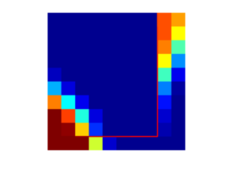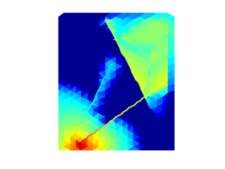# MRST - Transforming research on reservoir simulation

Discrete Fracture Matrix (DFM) Methods
This module implements control-volume methods for flow problems in fractured media for which the fractures are represented as lower-dimensional objects.

## Tutorials

###Basic Cartesian fracture grid

This script illustrates the basic use of the DFM module. We create a simple network of two fractures in a Cartesian 2D grid and add the fracuters to the grid with a hybrid representation. Transmissibilities are then computed with a two-point flux expression, and solve a tracer transport problem.

###Unstructured grid

A somewhat advanced example, where a fracture network is gridded with triangles using a fairly simple approach. The flow equations are then discretized with both two- and multi-point flux expressions, and a two-phase transport problem is solved.

## Description

The module provides control volume discretizations (two- and multi-point flux approximations) of fractured media by representing fractures as lower-dimensional objects that still are represented as cells in the computational grid. The discretization is described by Karimi-Fard et al.  and Sandve et al. . The approach assumes that the fractures are represented as faces in the grid, thus the module also provide some utility functions for triangulating fractured domains. Finally, the transport solvers (both explicit and implicit) from the incomp module are modified to account for fracture transport. MRST files that are modified have a suffix _DFM to distinguish them from the original files.

The module is mainly developed by Tor Harald Sandve (at IRIS from Jan 2013), minor modifications are introduced by Eirik Keilegavlen (University of Bergen). Some utility files are partly written at Uni Research. Technical details can be found in the implementation note.

## Literature

1. M. Karimi-Fard,  L. Durlofsky, and K. Aziz. An efficient discrete-fracture model applicable for general-purpose reservoir simulators. SPE Journal, 9(2):227-236, 2004. DOI: 10.2118/88812-PA
2. T.H. Sandve, and I. Berre, J. M. Nordbotten. An efficient multi-point flux approximation based approach for discrete fracture matrix simulation. Journal of Computational Physics, 231(9), 3784-3800, 2012. DOI:10.1016/j.jcp.2012.01.023.

Published December 8, 2016